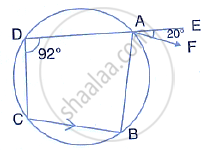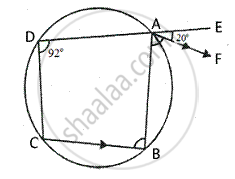Share

In the Given Figure, Abcd is a Cyclic Quadrilateral. Af is Drawn Parallel to Cb and Da is Produced to Point E. If ∠Adc = 92°, ∠Fae = 20°; Determine ∠Bcd. Give Reason in Support of Your Answer. - ICSE Class 10 - Mathematics

Question

In the given figure, ABCD is a cyclic quadrilateral. AF is drawn parallel to CB and DA is produced to point E. if ∠ADC = 92°, ∠FAE = 20°; determine ∠BCD. Give reason in support of your answer.SolutionIn cyclic quad. ABCD,
AF || CB and DA is produced to E such that ∠ADC = 92° and ∠FAE = 20°
Now we need to find the measure of ∠ ∠BCD
In cyclic quad. ABCD,
∠B + ∠ = 180°

⇒ ∠B + 92° = 180°
⇒ ∠B = 180° - 92°
⇒ ∠B = 88°
Since AF || CB, ∠FAB = ∠B = 88°
But, ∠FAE = 20°     [given]

Ext. ∠BAE = ∠BAF + ∠FAE
= 88° + 22° = 108°
But, Ext. ∠BAE = ∠BCD
∴ ∠BCD = 108°

Is there an error in this question or solution?
Solution In the Given Figure, Abcd is a Cyclic Quadrilateral. Af is Drawn Parallel to Cb and Da is Produced to Point E. If ∠Adc = 92°, ∠Fae = 20°; Determine ∠Bcd. Give Reason in Support of Your Answer. Concept: Cyclic Properties.
S